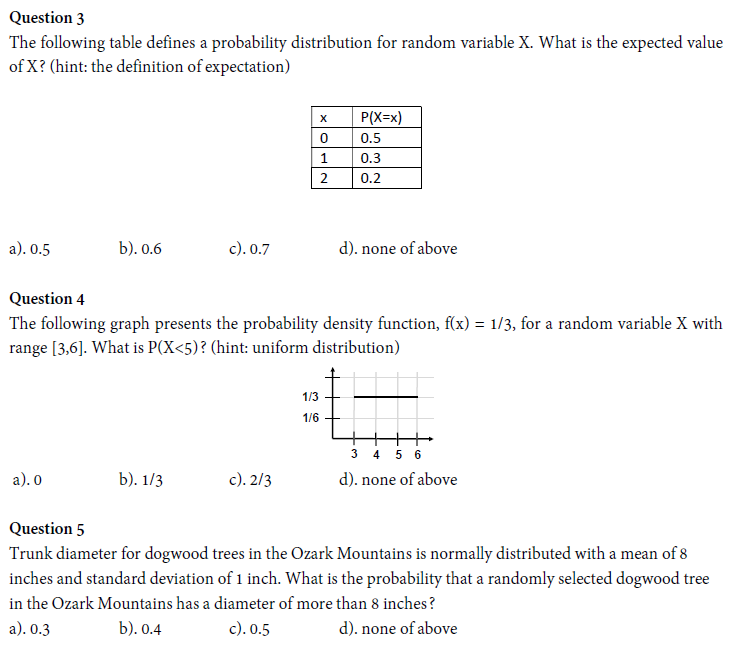# Question Question 3 The following table defines a probability distribution for random variable X. What is the expected value of X? (hint: the definition of expectation) x 0 1 2 P(X=x) 0.5 0.3 0.2 a). 0.5 b). 0.6 c).0.7 d). none of above Question 4 The following graph presents the probability density function, f(x) = 1/3, for a random variable X with range (3,6). What Question 3 The following table defines a probability distribution for random variable X. What is the expected value of X? (hint: the definition of expectation) x 0 1 2 P(X=x) 0.5 0.3 0.2 a). 0.5 b). 0.6 c).0.7 d). none of above Question 4 The following graph presents the probability density function, f(x) = 1/3, for a random variable X with range (3,6). What is P(X<5)? (hint: uniform distribution) 1/3 = 1/6 + 3 4 5 6 d). none of above a). b). 1/3 c). 2/3 Question 5 Trunk diameter for dogwood trees in the Ozark Mountains is normally distributed with a mean of 8 inches and standard deviation of 1 inch. What is the probability that a randomly selected dogwood tree in the Ozark Mountains has a diameter of more than 8 inches? a).0.3 b). 0.4 C).0.5 d). none of aboveL6URI6 The Asker · Probability and StatisticsTranscribed Image Text: Question 3 The following table defines a probability distribution for random variable X. What is the expected value of X? (hint: the definition of expectation) x 0 1 2 P(X=x) 0.5 0.3 0.2 a). 0.5 b). 0.6 c).0.7 d). none of above Question 4 The following graph presents the probability density function, f(x) = 1/3, for a random variable X with range (3,6). What is P(X<5)? (hint: uniform distribution) 1/3 = 1/6 + 3 4 5 6 d). none of above a). b). 1/3 c). 2/3 Question 5 Trunk diameter for dogwood trees in the Ozark Mountains is normally distributed with a mean of 8 inches and standard deviation of 1 inch. What is the probability that a randomly selected dogwood tree in the Ozark Mountains has a diameter of more than 8 inches? a).0.3 b). 0.4 C).0.5 d). none of above
More
Transcribed Image Text: Question 3 The following table defines a probability distribution for random variable X. What is the expected value of X? (hint: the definition of expectation) x 0 1 2 P(X=x) 0.5 0.3 0.2 a). 0.5 b). 0.6 c).0.7 d). none of above Question 4 The following graph presents the probability density function, f(x) = 1/3, for a random variable X with range (3,6). What is P(X<5)? (hint: uniform distribution) 1/3 = 1/6 + 3 4 5 6 d). none of above a). b). 1/3 c). 2/3 Question 5 Trunk diameter for dogwood trees in the Ozark Mountains is normally distributed with a mean of 8 inches and standard deviation of 1 inch. What is the probability that a randomly selected dogwood tree in the Ozark Mountains has a diameter of more than 8 inches? a).0.3 b). 0.4 C).0.5 d). none of above